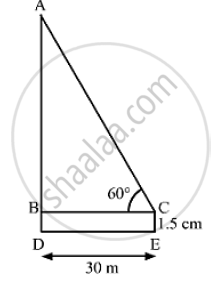# An Observer 1.5m Tall is 30 Away from a Chimney. the Angle of Elevation of the Top of the Chimney from His Eye is 60 . Find the Height of the Chimney. - Mathematics

An observer 1.5m tall is 30 away from a chimney. The angle of elevation of the top of the chimney from his eye is 60 . Find the height of the chimney.

#### SolutionLet CE and AD be the heights of the observer and the chimney, respectively.
We have,
BD = CE = 1.5 m, BC = DE = 30 mand ∠ACB = 60°
In ΔABC

tan 60° = (AB)/(BC)

⇒ sqrt(3 ) = (AD-BD)/30

⇒ AD -1.5 =30 sqrt(3)

⇒  AD = 30sqrt(3) +1.5

⇒  AD = 30XX 1.732 + 1.5

⇒  AD = 51.96 + 1.5

⇒  AD = 53.46 m

So, the height of the chimney is 53.46 m (approx).

Concept: Heights and Distances
Is there an error in this question or solution?
Chapter 14: Height and Distance - Exercises

#### APPEARS IN

RS Aggarwal Secondary School Class 10 Maths
Chapter 14 Height and Distance
Exercises | Q 3

Share